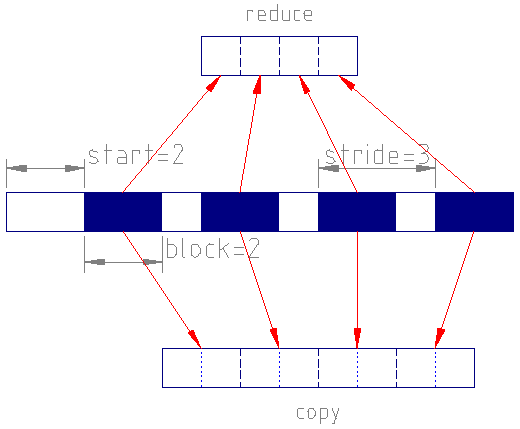# 3.3.3.47. NXregion¶

Status:

base class, extends NXobject

Description:

Geometry and logical description of a region of data in a parent group. When used, it could be a child group to, say, NXdetector.

This can be used to describe a subset of data used to create downsampled data or to derive some data from that subset.

Note, the fields for the rectangular region specifiers follow HDF5’s dataspace hyperslab parameters (see https://portal.hdfgroup.org/display/HDF5/H5S_SELECT_HYPERSLAB). Note when block $$= 1$$, then stride $$\equiv$$ step in Python slicing.

For example, a ROI sum of an area starting at index of [20,50] and shape [220,120] in image data:

detector:NXdetector/
data[60,256,512]
region:NXregion/
@region_type  = "rectangular"
parent = "data"
start  = [20,50]
count  = [220,120]
statistics:NXdata/
@signal = "sum"
sum


the sum dataset contains the summed areas in each frame. Another example, a hyperspectral image downsampled 16-fold in energy:

detector:NXdetector/
data[128,128,4096]
region:NXregion/
@region_type  = "rectangular"
parent = "data"
start  = 
count  = 
stride = 
block  = 
downsampled:NXdata/
@signal = "maximum"
@auxiliary_signals = "copy"
maximum[128,128,20]
copy[128,128,320]


the copy dataset selects 20 16-channel blocks that start 32 channels apart, the maximum dataset will show maximum values in each 16-channel block in every spectra.

Symbols:

These symbols will denote how the shape of the parent group’s data field,

$(D_0, ..., D_{\mathbf{O}-1}, d_0, ..., d_{\mathbf{R}-1})$

could be split into a left set of O outer dimensions, $$\boldsymbol{D}$$, and a right set of R region dimensions, $$\boldsymbol{d}$$, where the data field has rank O + R. Note O $$>= 0$$.

O: Outer rank

R: Region rank

Groups cited:

NXdata

Structure:

@region_type: (required) NX_CHAR

This is rectangular to describe the region as a hyper-rectangle in the index space of its parent group’s data field.

Obligatory value: rectangular

parent: (optional) NX_CHAR

The name of data field in the parent group or the path of a data field relative to the parent group (so it could be a field in a subgroup of the parent group)

The name of an optional mask field in the parent group with rank $$\boldsymbol{R}$$ and dimensions $$\boldsymbol{d}$$. For example, this could be pixel_mask of an NXdetector.

start: (recommended) NX_NUMBER (Rank: 1, Dimensions: [R])

The starting position for region in detector data field array. This is recommended as it also defines the region rank. If omitted then defined as an array of zeros.

count: (recommended) NX_INT (Rank: 1, Dimensions: [R])

The number of blocks or items in the hyperslab selection. If omitted then defined as an array of dimensions that take into account the other hyperslab selection fields to span the parent data field’s shape.

stride: (optional) NX_INT (Rank: 1, Dimensions: [R])

An optional field to define striding used to downsample data. If omitted then defined as an array of ones.

block: (optional) NX_INT (Rank: 1, Dimensions: [R])

An optional field to define the block size used to copy or downsample data. In the $$i$$-th dimension, if $$\mathbf{block}[i] < \mathbf{stride}[i]$$ then the downsampling blocks have gaps between them; when block matches stride then the blocks are contiguous; otherwise the blocks overlap. If omitted then defined as an array of ones.

scale: (optional) NX_NUMBER (Rank: 1, Dimensions: [R])

An optional field to define a divisor for scaling of reduced data. For example, in a downsampled sum, it can reduce the maximum values to fit in the domain of the result data type. In an image that is downsampled by summing 2x2 blocks, using $$\mathrm{scale}=4$$ allows the result to fit in the same integer type dataset as the parent dataset. If omitted then no scaling occurs.

downsampled: (optional) NXdata

An optional group containing data copied/downsampled from parent group’s data. Its dataset name must reflect how the downsampling is done over each block. So it could be a reduction operation such as sum, minimum, maximum, mean, mode, median, etc. If downsampling is merely copying each block then use “copy” as the name. Where more than one downsample dataset is written (specified with @signal) then add @auxiliary_signals listing the others. In the copy case, the field should have a shape of $$(D_0, ..., D_{\mathbf{O}-1}, \mathbf{block} * \mathbf{count}, ..., \mathbf{block}[\mathbf{R}-1] * \mathbf{count}[\mathbf{R}-1])$$, otherwise the expected shape is $$(D_0, ..., D_{\mathbf{O}-1}, \mathbf{count}, ..., \mathbf{count}[\mathbf{R}-1])$$.

The following figure shows how blocks are used in downsampling:A selection with $$\mathbf{start}=2, \mathbf{count}=4, \mathbf{stride}=3, \mathbf{block}=2$$ from a dataset with shape  will result in the reduce dataset of shape  and a copy dataset of shape .¶

statistics: (optional) NXdata

An optional group containing any statistics derived from the region in parent group’s data such as sum, minimum, maximum, mean, mode, median, rms, variance, etc. Where more than one statistical dataset is written (specified with @signal) then add @auxiliary_signals listing the others. All data fields should have shapes of $$\boldsymbol{D}$$.

## Hypertext Anchors¶

List of hypertext anchors for all groups, fields, attributes, and links defined in this class.

NXDL Source:

https://github.com/nexusformat/definitions/blob/main/contributed_definitions/NXregion.nxdl.xml